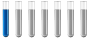## Physical chemistry

Chemistry and homework help forum.

Organic Chemistry, Analytical Chemistry, Biochemistry, Physical Chemistry, Computational Chemistry, Theoretical Chemistry, High School Chemistry, Colledge Chemistry and University Chemistry Forum.

Share your chemistry ideas, discuss chemical problems, ask for help with scientific chemistry questions, inspire others by your chemistry vision!

Please feel free to start a scientific chemistry discussion here!

Discuss chemistry homework problems with experts!

Ask for help with chemical questions and help others with your chemistry knowledge!

Moderators: Xen, expert, ChenBeier

Max123
NewbiePosts: 1
Joined: Tue Sep 05, 2023 8:46 am

### Physical chemistry

Hello!

I need to create phase diagram (P-T) using PC-SAFT equation. Which parameters I have to calculate to do this?

Is it correct that I should equate the fugitivities of the components in both phases and if the equation has a solution then they exist, if not then the system is single phase?
Weser
NewbiePosts: 1
Joined: Sun Sep 24, 2023 9:29 am

### Re: Physical chemistry

Max123 wrote: Tue Sep 05, 2023 8:50 am Hello!

I need to create phase diagram (P-T) using PC-SAFT equation. Which parameters I have to calculate to do this?

Is it correct that I should equate the fugitivities of the components in both phases and if the equation has a solution then they exist, if not then the system is single phase?
Formulation of PC-SAFT: Start by formulating the PC-SAFT equation for your specific system. This will involve defining the segment numbers, chain lengths, and molecular parameters for your components.
Equilibrium Conditions: To determine phase equilibria, you're correct that you should equate the fugacities of the components in both phases. The fugacity is a measure of the escaping tendency of a component from the mixture. For a two-phase equilibrium, you'd equate the fugacities of each component in the liquid and vapor phases.
Gibbs-Duhem Equation: Apply the Gibbs-Duhem equation to relate changes in pressure, temperature, and composition for coexisting phases. This equation helps in calculating the phase equilibrium conditions.
Parameter Estimation: You may need to estimate or obtain PC-SAFT parameters for your specific components, which can involve experimental data or theoretical calculations.
Numerical Methods: Solve the equations numerically. Various numerical methods like Gibbs energy minimization or flash calculations may be used.
Construction of Phase Diagram: With the obtained data, construct your P-T phase diagram. Plotting pressure against temperature while varying composition will reveal phase boundaries and regions of single-phase and two-phase regions.
Validation: Validate your results against experimental data if available to ensure the accuracy of your phase diagram.
jesse99
NewbiePosts: 1
Joined: Mon Oct 02, 2023 8:19 pm
Location: https://wordleunlimited.online/travle

### Re: Physical chemistry

You must identify the components, figure out their PC-SAFT parameters, and compare the component fugacities in both phases at various temperature (T) and pressure (P) points to determine phase equilibrium in order to build a phase diagram (P-T) using the PC-SAFT equation.